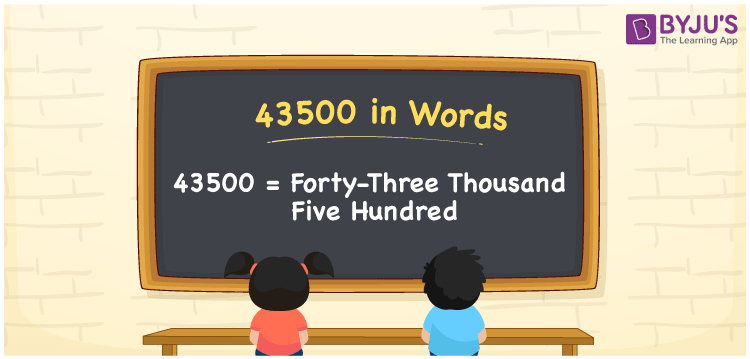# 43500 in words

43500 in words is written as Forty Three Thousand Five Hundred. In 43500, 4 has a place value of ten thousand, 3 has a place value of thousand and 5 has a place value of hundred. The article on Place Value gives more information. The number 43500 is used in expressions that relate to money, distance, length, count and many more. For example, “My new mobile cost me Forty Three Thousand Five Hundred Rupees.” Another example. “For a fitness challenge, I took 30 days to cover Forty Three Thousand Five Hundred steps”.

 43500 in words Forty Three Thousand Five Hundred Forty Three Thousand Five Hundred in Numbers 43500

## 43500 in English Words## How to Write 43500 in Words?

We can convert 43500 to words using a place value chart. The number 43500 has 5 digits, so let’s make a chart that shows the place value up to 5 digits.

 Ten thousand Thousands Hundreds Tens Ones 4 3 5 0 0

Thus, we can write the expanded form as:

4 × Ten thousand + 3 × Thousand + 5 × Hundred + 0 × Ten + 0 × One

= 4 × 10000 + 3 × 1000 + 5 × 100 + 0 × 10 + 0 × 1

= 43500

= Forty Three Thousand Five Hundred.

43500 is the natural number that is succeeded by 43499 and preceded by 43501.

43500 in words – Forty Three Thousand Five Hundred.

Is 43500 an odd number? – No.

Is 43500 an even number? – Yes.

Is 43500 a perfect square number? – No.

Is 43500 a perfect cube number? – No.

Is 43500 a prime number? – No.

Is 43500 a composite number? – Yes.

## Solved Example

1. Write the number 43500 in expanded form

Solution: 4 x 10000 + 3 x 1000 + 5 x 100 + 0 x 10 + 0 x 1.

Or Just 4 x 10000 + 3 x 1000 + 5 x 100.

We can write 43500 =40000 + 3000 + 500 + 0 + 0

= 4 x 10000 + 3 x 1000 + 5 x 100 + 0 x 10 + 0 x 1.

## Frequently Asked Questions on 43500 in words

Q1

### How to write 43500 in words?

43500 in words is written as Forty Three Thousand Five Hundred.
Q2

### State whether True or False. 43500 is divisible by 3?

True. 43500 is divisible by 3.
Q3

### Is 43500 divisible by 10?

Yes. 43500 is divisible by 10.